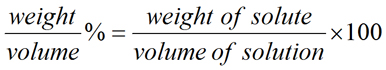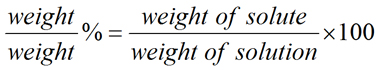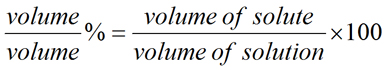PhysiologyWeb  Home  |  FAQ  |  About  |  Contact  |   - Sign InTweetPercent (%) Solutions Calculator
Meant to be used in both the teaching and research laboratory, this calculator (see below) can be utilized to perform a number of different calculations for preparing percent (%) solutions when starting with the solid or liquid material. It is very common to express the concentration of solutions in terms of percentages. Percent means per 100 parts, where for solutions, part refers to a measure of mass (μg, mg, g, kg, etc.) or volume (μL, mL, L, etc.). In percent solutions, the amount (weight or volume) of a solute is expressed as a percentage of the total solution weight or volume. Percent solutions can take the form of weight/volume % (wt/vol % or w/v %), weight/weight % (wt/wt % or w/w %), or volume/volume % (vol/vol % or v/v %). In each case, the percentage concentration is calculated as the fraction of the weight or volume of the solute related to the total weight or volume of the solution.
Because percent solutions can be expressed in three different ways, it is imperative that the type of percent solution be explicitly stated. If this information is not provided, the end user is left to "guess" whether w/v %, w/w %, or v/v % was used. Each percent solution is appropriate for a number of different applications. For example, commercial aqueous reagents, such as concentrated acids and bases, are typically expressed as weight/weight % solutions. For example, commercially available concentrated hydrochloric acid (HCl) is 37% by weight (w/w %). On the other hand, many dilute solutions used for biological research are expressed as weight/volume % (e.g., 1% sodium dodecyl sulfate, SDS). Volume/volume % solutes are also common, and are used when pure solutes in liquid form are used. For example, a 70 % (v/v) solution of ethanol can be prepared by dissolving 70 mL of 100% (i.e., 200 proof) ethanol in a total solution volume of 100 mL.
Other factors may also be important when deciding on the type of percent solution to prepare. For example, if the percent solution under consideration is to be used at widely different temperatures, then it is better to prepare the solution as a weight/weight % solution because its concentration would be independent of variations in ambient temperature.
An important note is in order. Here, we have used "weight" instead of "mass" simply to be consistent with tradition and popular use. Thus, weight/volume % solutions should be correctly referred to as mass/volume %. Similarly, weight/weight % solutions should be referred to as mass/mass %, or simply mass %.
If you wish to perform dilution calculations for solutions with molarity or percent concentration units, use our Dilution Calculator - Molarity, Percent.
If you wish to perform dilution factor or fold dilution calculations for solutions with molarity or percent concentration units, use our Dilution Factor Calculator - Molarity, Percent.
Percent solution equations(Eq. 1)(Eq. 2)(Eq. 3)

As noted above, weight refers to mass (i.e., measured on a balance). When examining the equation for each of the percent solutions above, it is very important to note that in all cases the denominator refers to the solution mass or volume and not just the solvent mass or volume. Thus, solution mass is the combined mass of solute and solvent, and solution volume is the combined volume of solute and solvent.
A final note is necessary when considering volume/volume % solutions. When different volumes of an identical solution are added together, the final volume will always be exactly the sum of the individual portions added. For example, adding 50 mL of water to 50 mL of water will result in a total volume of 100 mL, and adding 75 mL of 100% ethanol to 75 mL of 100% ethanol will result in a total volume of 150 mL. However, when mixing miscible liquids (such as water and ethanol), the final volume of solution is not exactly equal to the sum of the individual volumes. For example, adding 50 mL of ethanol to 50 mL of water will result in a total volume that is less than 100 mL. It is actually closer to 96 mL. Therefore, when preparing volume/volume percent solutions, it is always better to dissolve the solute in solvent and then add additional solvent to bring the total solution volume to the desired final value.
Percent Solution Calculator
Each calculator cell shown below corresponds to a term in the formula presented above. Enter appropriate values in all cells except the one you wish to calculate. Therefore, at least two cells must have values, and no more than one cell may be blank. The value of the blank cell will be calculated based on the other values entered. After a calculation is performed, the calculated cell will be highlighted and subsequent calculations will calculate the value of the highlighted cell (with no requirement to have a blank cell). However, a blank cell has priority over a highlighted cell.